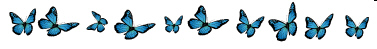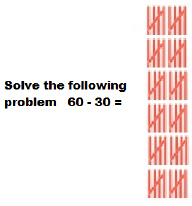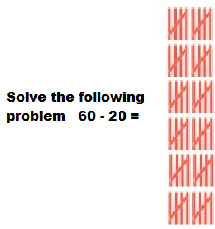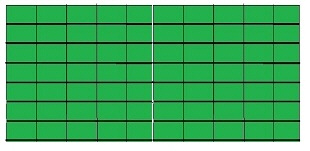Name:    1NBT6

 CompletionComplete each statement. 1.Solve the following problem. 20 -10= 2.Solve the following problem. 50-30= 3.Solve the following problem.  30-20= 4.Solve this equation:  10 - 10 = ? 5.Solve the following problem. 40 -30= 6.7.8.Solve the following problem.  70-40 = 9.Solve the following problem.  90-20 = 10.Solve the following problem.  90-40=